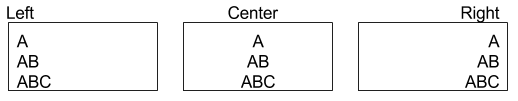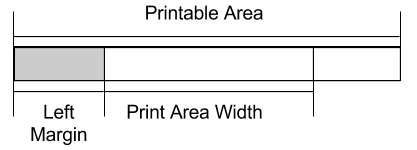# Layout Commands¶

This section describes all commands that affect the layout of text in terms of spacing and margins. These are advanced features that are not commonly by most users.

## Right Side Character Spacing - $1B$20¶

Sets the right-side character spacing to [n × (horizontal or vertical motion unit)].

Format: Hex       $1B$20 n ASCII     ESC SP n Decimal   27  32 n Settings that exceed the printable area are ignored. The maximum right side character spacing is 255/204 inches. The horizontal (perpendicular to paper feed) motion unit is used. The horizontal and vertical motion units are specified by Motion Units. Changing the horizontal or vertical motion unit does not affect the current right-side character spacing. Right-Side character spacing is effective until it is changed, Initialize is executed, the printer is reset, or the power is turned off. When underline mode is turned on, the right side character spacing is underlined. In standard mode, right side character spacing has no effect when characters are rotated 90​°​ or 270​°. 0 ≤ n ≤ 255 0 Motion Units Relative Print Position None

## Line Spacing - $1B$33¶

Sets the line spacing to [n (vertical or horizontal motion unit)] in inches

Format: Hex       $1B$33 n ASCII     ESC 3 n Decimal   27  51 n This command sets the line spacing in standard mode. The vertical and horizontal motion units are specified by Motion Units. Changing the vertical or horizontal motion units does not affect the current line spacing. If the calculation results in a fraction, the decimal portion will be ignored. The vertical motion unit is used. Minimum line spacing = 0.00492 inches (0.125mm) Maximum line spacing = 4 inches (101.6mm) Line spacing is effective until it is changed by another command, Initialize is executed, the printer is reset, or the power is turned off. 0 ≤ n ≤ 255 34 (1/6"), n is base 10 Motion Units Line Spacing Select 1/6” Line Spacing Select 1/8” Line Spacing None

## Select 1/6 Inch Line Spacing - $1B$32¶

Sets the line spacing to 1/6 an inch

Format:

Hex       $1B$32

ASCII     ESC 2

Decimal   27  50

Notes:
• This command sets the line spacing in standard mode.
• Line spacing is effective until it is changed by another command, Initialize is executed, the printer is reset, or the power is turned off.
Range:

None

Default:

None

Related:
Example: None

## Select 1/8 Inch Line Spacing - $1B$30¶

Sets the line spacing to 1/8 an inch
Format: Hex       $1B$30 ASCII     ESC 0 Decimal   27  48 This command sets the line spacing in standard mode. Line spacing is effective until it is changed by another command, Initialize is executed, the printer is reset, or the power is turned off. None None 1/6 inch spacing Motion Units Line Spacing None

## Select Justification - $1B$61¶

Select justification mode

Format: Hex       $1B$61 n ASCII     ESC a   n Decimal   27  97  n This command is enabled only when processed at the beginning of a line. (When the current line is empty) This command applies the justification within the area set by Left Margin ​and Print Area Width This command will justify all data in the printing area such as characters, graphics, bit images, barcode and space area set by Horizontal Tab, Absolute and Relative print positions. Settings of this command are effective until ​the Initialize command is executed, the printer is reset, or the power is turned off. When n=0 or 48, left justification is enabled When n=1 or 49, center justification is enabled When n=2 or 50, right justification is enabled n=0,1,2,48,49,50 0 Left Margin Print Area Width Absolute Print Position Relative Print Position Horizontal Tab## Left Margin - $1D$4C¶

Set left margin
Format: Hex       $1D$4C nL nH ASCII     GS  L   nL nH Decimal   27  76  nL nH In standard mode, sets the left margin to [(nL + (nH × 256)) × (horizontal motion unit)] from the left edge of the printable area. Uses Two Byte Number Definitions. This command is enabled only when processed at the beginning of a line. (When the current line is empty) This command applies the justification within the area set by Left Margin ​and Print Area Width This command will justify all data in the printing area such as characters, graphics, bit images, bar code and space area set by Horizontal Tab, Absolute and Relative print positions. Settings of this command are effective until Initialize is executed, the printer is reset, or the power is turned off.0 ≤ nL, nH ≥ 255, 0 ≤ (nL + (nH × 256))≤ 65535 nL = 0, nH =0 Motion Units Print Area Width None

## Motion Units - $1D$50¶

Set horizontal and vertical motion units
Format: Hex       $1D$50 x   y ASCII     GS  P   x   y Decimal   29  80  x   y Sets the horizontal and vertical motion units to approximately 25.4/x mm {1/x”} and approximately 25.4/y mm {1/y”}, respectively. When x = 0, the default value of the horizontal motion unit is used. When y = 0, the default value of the vertical motion unit is used. When x > 204, the default value of the horizontal motion unit is used. When y > 204, the default value of the vertical motion unit is used. The horizontal direction is perpendicular to the paper feed direction and the vertical direction is the paper feed direction. The horizontal and vertical motion units indicate the minimum pitch used for calculating the values of related commands In standard mode, the following commands use x or y. Commands using x: Left Margin, Print Area Width Commands using y If the result is a decimal number, the decimal is ignored. This command does not affect the previously defined values for settings that use the horizontal or vertical motion units. Settings of this command are effective until it is changed, Initialize is executed, the printer is reset, or the power is turned off. 0 ≤ x, y ≤ 204 x = 204, y = 204 Left Margin Print Area Width None